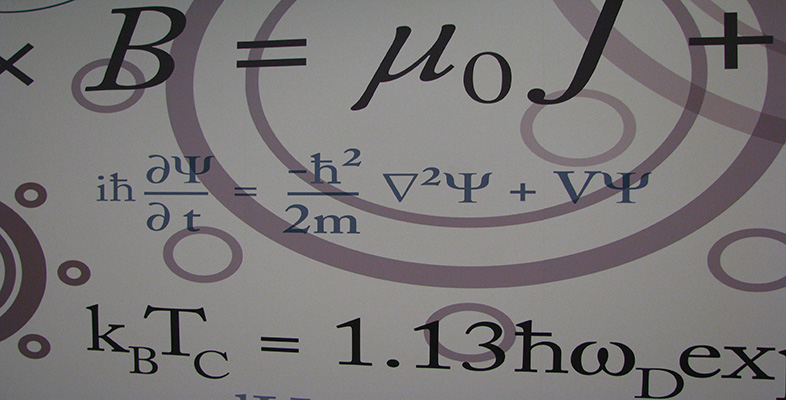Number systems

This free course is available to start right now. Review the full course description and key learning outcomes and create an account and enrol if you want a free statement of participation.

Free course

2.6 Division of complex numbers

The second of the conjugate–modulus properties enables us to find reciprocals of complex numbers and to divide one complex number by another, as shown in the next example. As for real numbers, we cannot find a reciprocal of zero, nor divide any complex number by zero.

Example 2

• (a)  Find the reciprocal of 2 − 5i.

• (b)  Find the quotientSolution

• (a)  We want to find the complex number which represents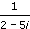• We multiply the numerator and denominator by 2 + 5i, the complex conjugate of 2 − 5i, to give

•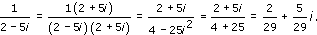• (b)  We multiply the numerator and denominator by 1 −2i, the complex conjugate of 1 + 2i, to give

•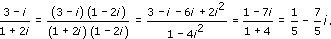The method used in Example 2, of multiplying the numerator and denominator by the complex conjugate of the denominator, enables us to find the reciprocal of any non-zero complex number z, and the quotient z1/z2 of any two complex numbers z1 and z2, where z2 ≠ 0. We can obtain general formulas as follows.

For the reciprocal, we have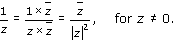If z = x + iy, so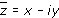and |z|2= x2 + y2, we obtain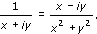For the quotient z1/z2, we have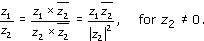If z1 = x1 + iy1 and z2 = x2 + iy2, this can be rewritten as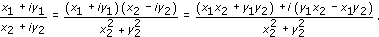These formulas may be used in theoretical work, but for calculations of reciprocals and quotients it is simplest to use the method of Example 2.

Exercise 13

Find the reciprocal of each of the following complex numbers.

• (a)  3 − i

• (b)  −1 + 2i

Solution

In each case we multiply both the numerator and the denominator by the complex conjugate of the denominator.

• (a)

•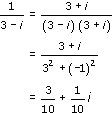• (b)

•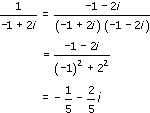Exercise 14

Evaluate each of the following quotients.

• (a)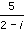• (b)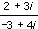Solution

In each case we multiply the numerator and denominator by the complex conjugate of the denominator.

• (a)

•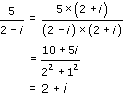• (b)

•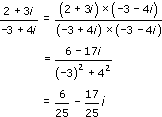M208_6